four

💎 A 4-dimensional renderer.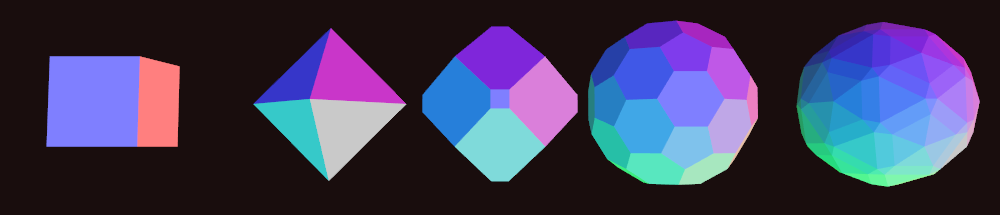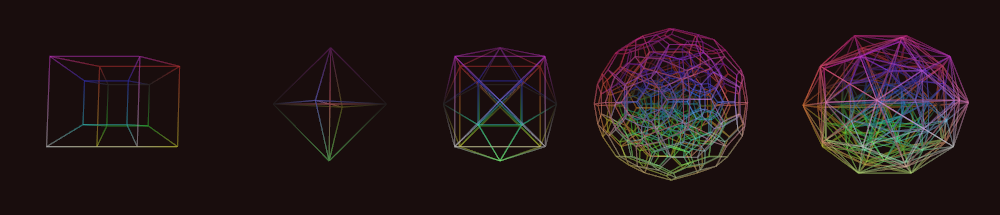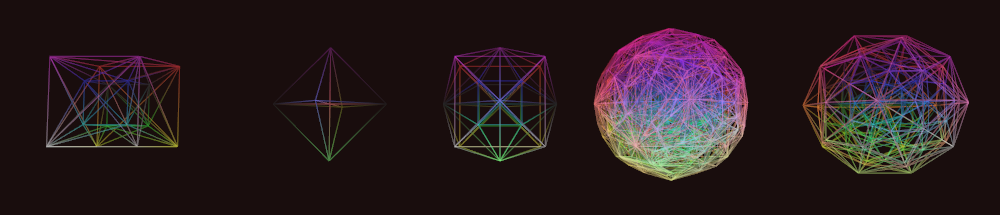Description

Projections

After seeing videos of Miegakure gameplay, I became very interested in the idea of 4-dimensional rendering. It turns out that there are several ways to visualize higher dimensional objects. Perhaps the simplest method involves a projection from 4D to 3D. This is similar to how "traditional" 3D engines render objects to a 2D display surface (your screen). A 4D -> 3D projection can either be a perspective or parallel (orthographic) projection. However, instead of projecting objects onto a flat plane (as is the case in a 3D -> 2D projection), we project our 4-dimensional objects "onto" a 3-dimensional cube (in this case, the unit cube centered at the origin). From here, we simply perform the 3D -> 2D projection that we are used to.

Most people are familiar with this form of visualization. When a 4D -> 3D perspective projection is applied to the 8-cell (also known as the hypercube or tesseract), the result is the familiar animation of an "outer" cube with a smaller, nested "inner" cube that appears to unfold itself via a series of 4-dimensional plane rotations. The reason why this inner cube appears smaller is because it is "further away" in the 4th dimension (the w-axis of our 4D coordinate system). If you are interested in this form of visualization, I highly recommend Steven Hollasch's thesis, titled Four-Space Visualization of 4D Objects.

Slicing

A different way to visualize 4-dimensional objects is via a "slicing" procedure, which produces a series of 3-dimensional cross-sections of the full 4-dimensional shape. This is analogous to cutting 3D polyhedra with a plane (think MRI scans). Luckily, much of the math carries over to 4D. In order to facilitate this process, meshes in four are first decomposed into a set of tetrahedrons. This is similar to how we decompose the faces of a regular polyhedron into triangles ("triangulation"). In particular, any 3D convex polyhedron can be decomposed into tetrahedrons by first subdividing its faces into triangles. Next, we pick a vertex from the polyhedron (any vertex will do). We connect all of the other face triangles to the chosen vertex to form a set of tetrahedra (obviously, ignoring faces that contain the chosen vertex). This is not necessarily the "minimal tetrahedral decomposition" of the polyhedron (which is an active area of research for many polytopes), but it always works. An example of this process for a regular, 3D cube can be found here.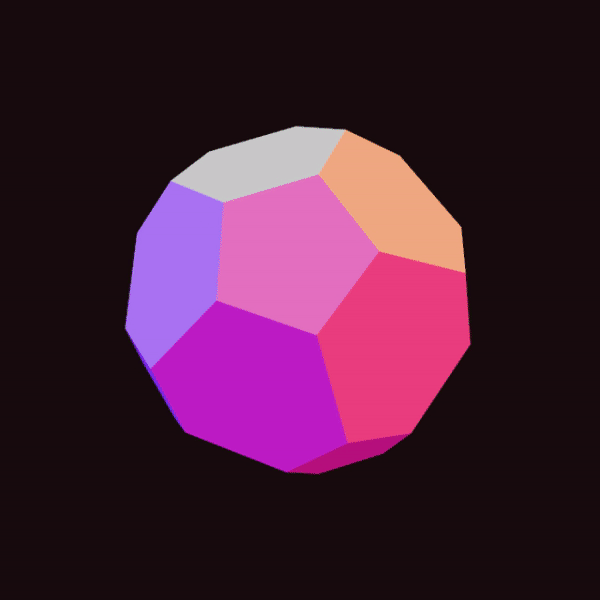So, we start with our 4D polychoron, whose "faces" (usually referred to as "cells") are themselves convex polyhedra embedded in 4-dimensions. One by one, we "tetrahedralize" each cell, and together, the sum of these tetrahedra form our 4-dimensional mesh. For example, the hypercube has 8 cells, each of which is a cube (this is why the hypercube is called the 8-cell). Each cube produces 6 distinct tetrahedra, so all together, the tetrahedral decomposition of the hypercube results in 48 tetrahedra. This process can be seen below for an icosahedron: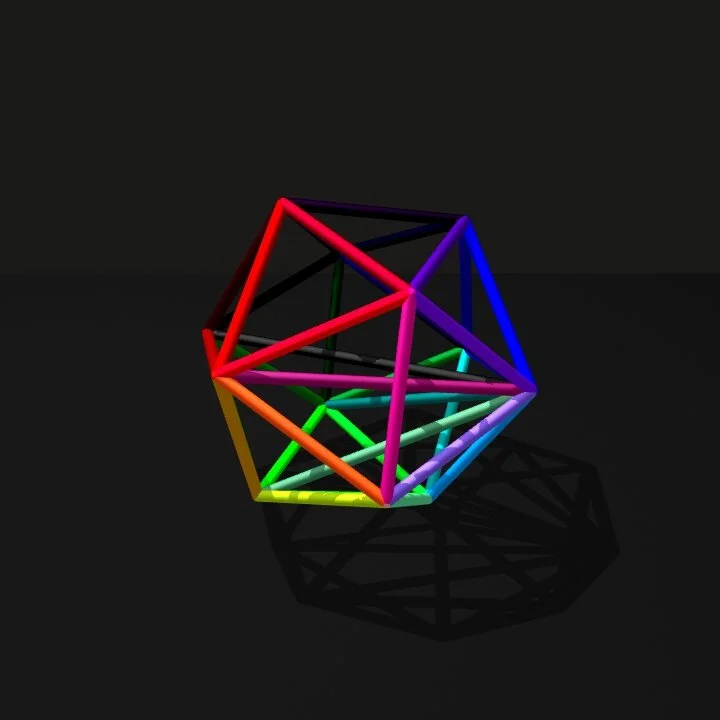Why do we do this? It turns out that slicing a tetrahedron in 4-dimensions is much simpler than slicing the full cells that make up the surface of a polytope. In particular, a sliced tetrahedron (embedded in 4-dimensions) will always produce zero, 3, or 4 vertices, which makes things quite a bit easier (particularly, when it comes to computing vertex indices for OpenGL rendering).

Implementation of the Slicing Procedure

Each mesh in four maintains a GPU-side buffer that holds all of its tetrahedra (each of which is an array of 4 vertices). The slicing operation is performed via a compute shader that ultimately generates a new set of vertices (the "slice") for each tetrahedron. The same compute shader also generates draw commands on the GPU, which are later dispatched via glMultiDrawArraysIndirect. Essentially, each tetrahedron will generate its own unique draw command that renders either 0, 1, or 2 triangles, depending on whether the slicing operation returned an empty intersection (0), a single triangle (1), or a quad (2).

In the case where a tetrahedron's slice is a quad, care needs to be taken in order to ensure a proper vertex winding order. This too is handled in the compute shader: the 4 vertices are sorted based on their signed angle with the polygon's normal. This is accomplished via a simple insertion sort. In GLSL, this looks something like:

uint i = 1;
while(i < array.length())
{
uint j = i;
while(j > 0 && (array[j - 1] > array[j]))
{
vec2 temp = array[j];
array[j] = array[j - 1];
array[j - 1] = temp;

j--;
}
i++;
}

Tested On

• Windows 8.1, Windows 10, Ubuntu 18.04
• NVIDIA GeForce GTX 970M, NVIDIA GeForce GTX 980
• Rust compiler version 1.37.0-nightly (nightly may not be required)

NOTE: this project will only run on graphics cards that support OpenGL Direct State Access (DSA).

To Build

1. Clone this repo.
2. Make sure 🦀 Rust installed and cargo is in your PATH.
3. Inside the repo, run: cargo build --release.

To Use

four currently supports all 5 regular 4-polytopes: 8-cell, 16-cell, 24-cell, 120-cell, and the 600-cell (the 5-cell or simplex is currently omitted).

To rotate the camera around the object in 3-dimensions, press + drag the left mouse button (this part definitely needs some refinement!). You can zoom the camera in or out using the scroll wheel.

There are 6 possible plane rotations in a 4-dimensional space (see math.rs for more details), and I haven't found a great way to expose this to the user (yet). For now, you can hold shift while pressing + dragging the left mouse button to rotate in the XW or YW planes. Alternatively, you can hold ctrl while pressing + dragging the left mouse button to rotate in the XY or ZX planes. You can change the "height" of the slicing hyperplane (effectively adjusting the w-coordinate of its "normal" vector) by pressing + dragging the right mouse button (without any modifiers).

You can change between wireframe and filled modes by pressing w and f.

Finally, you can toggle between 3 different projections / draw "modes" by repeatedly pressing t:

1. Slices: show the 3-dimensional slice of each polychoron, as dictated by the aforementioned "slicing hyperplane"
2. Tetrahedral wireframes: show the 3-dimensional projection of the 4-dimensional tetrahedral decomposition of each polychoron
3. Skeleton: show the 3-dimensional projection of the wireframe of the 4-dimensional polychoron

All of the draw modes listed above will be affected by the 4-dimensional rotations mentioned prior.

To Do

• [x] Implement a more generic approach to deriving a polytope's H-representation based on its dual
• [x] Finish additional polytopes (600-cell, etc.)
• [ ] Add 4-dimensional "extrusions" (i.e. things like spherinders)
• [ ] Add "hollow"-cell variants of each polytope (see Miegakure)
• [ ] Research 4-dimensional knots, un-tying, un-boxing, etc.
• [ ] Add simple lighting (calculate 3D normals after slicing procedure)
• [ ] Research Munsell color solids

Credits

The majority of the shape definitions in the polychora module are from Paul Bourke's website. Eventually, these shapes will (hopefully) be generated procedurally, but for now, they are hardcoded as Vec<T>s. Note that the vertices are slightly modified from the original source: in particular, all vertices are "normalized" to have unit length.

Thanks to @GSBicalho and @willnode for their guidance throughout this project. Their responses to my questions were vital towards my understanding the 4D slicing procedure.

I would like to give a special "thank you" to the author of Eusebeia, "T", who answered many, many of my (probably stupid) questions, provided a more accurate .txt file for the 120-cell, and helped me understand much of the necessary math that was required in order to complete this project. If you are interested in higher-dimensional rendering, I would encourage you to check out their site!